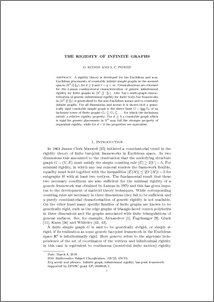# The rigidity of infinite graphs

Kitson, D. and C. Power, S. (2018) The rigidity of infinite graphs. Discrete and Computational Geometry, 60 (3). pp. 531-557. ISSN 0179-5376Preview
PDF (The_rigidity_of_infinite_graphs)
The_rigidity_of_infinite_graphs.pdf - Accepted Version

## Abstract

A rigidity theory is developed for the Euclidean and non-Euclidean placements of countably infinite simple graphs in the normed spaces $(\bR^d,\|\cdot \|_q)$, for $d\geq 2$ and $1 <q < \infty$. Generalisations are obtained for the Laman combinatorial characterisation of generic infinitesimal rigidity for  finite graphs in  $(\bR^2,\|\cdot \|_2)$.  Also Tay's multi-graph characterisation of generic infinitesimal rigidity for finite body-bar frameworks in $(\bR^d,\|\cdot\|_2)$ is generalised to the non-Euclidean norms and to countably infinite graphs. For all dimensions and norms  it is shown that a generically rigid countable simple graph is the direct limit $G= \varinjlim G_k$ of an inclusion tower of finite graphs $G_1 \subseteq G_2 \subseteq \dots$ for which the inclusions satisfy a relative rigidity property. For $d\geq 3$ a countable graph which is rigid for generic placements in  $\bR^d$ may fail the stronger property of  sequential rigidity, while for $d=2$ the properties are equivalent.

Item Type:
Journal Article
Journal or Publication Title:
Discrete and Computational Geometry
The final publication is available at Springer via http://dx.doi.org/10.1007/s00454-018-9993-0
Uncontrolled Keywords:
/dk/atira/pure/subjectarea/asjc/2600/2607
Subjects:
?? math.mgmath.co52c25, 05c63discrete mathematics and combinatoricscomputational theory and mathematicsgeometry and topologytheoretical computer science ??
Departments:
ID Code:
123994
Deposited By:
Deposited On:
09 Mar 2018 16:42
Refereed?:
Yes
Published?:
Published